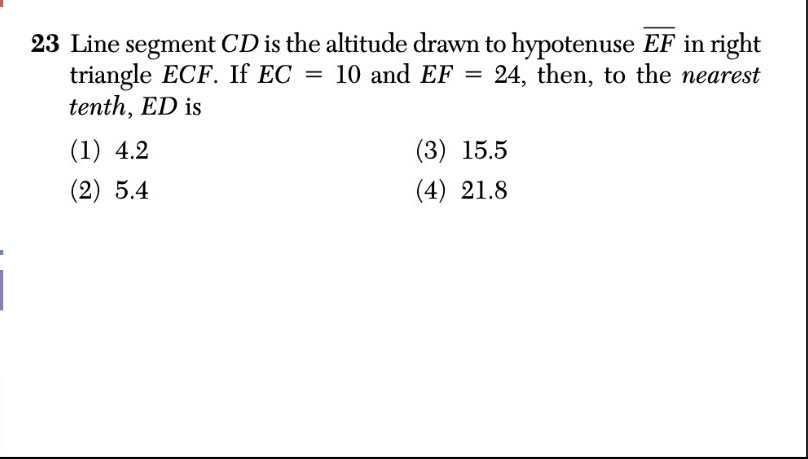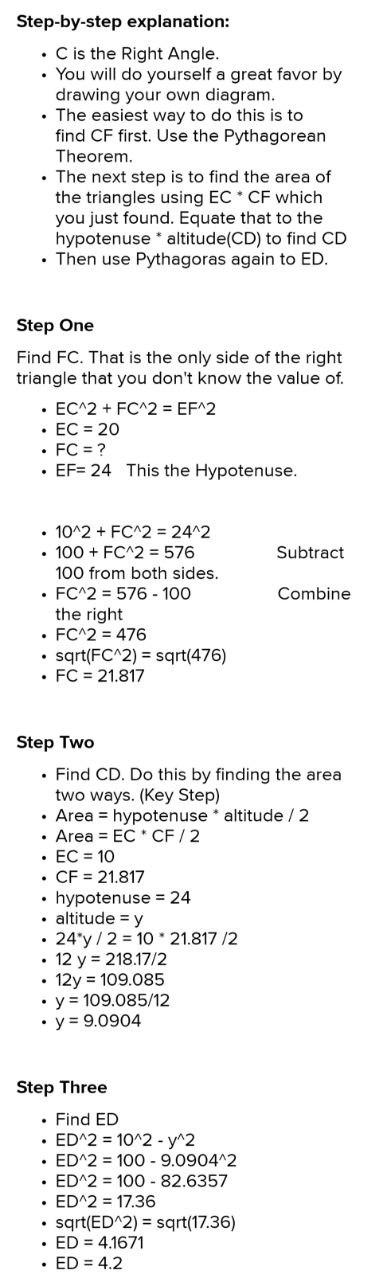### Still have math questions?

Trigonometry
QuestionLine segment $$C D$$ is the altitude drawn to hypotenuse $$\overline { E F }$$ in right triangle $$E C F$$ . If $$E C = 10$$ and $$E F = 24$$ , then, to the nearest tenth, $$E D$$ is

(1) $$4.2$$

(3) $$15.5$$

(2) $$5.4$$

(4) $$21.8$$